## 《高等数学》21春平时作业1

《高等数学》21春平时作业1

------------------------
1.在同一变化过程中，无穷小与无穷大的乘积是（ ）
A.无穷大
B.无穷小
C.非零常数
D.不能确定

2.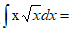（ ）
A.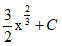B.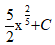C.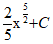D.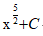3.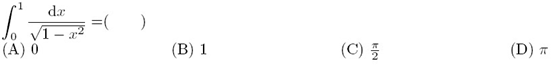A.(A)
B.(B)
C.(C)
D.(D)

4.设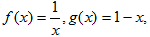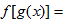（ 　）
A.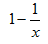B.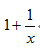C.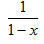D.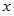5.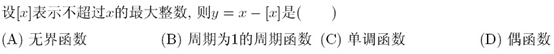A.(A)
B.(B)
C.(C)
D.(D)

6.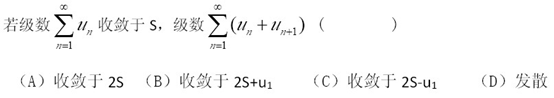A.(A)
B.(B)
C.(C)
D.(D)

7.A.(A)
B.(B)
C.(C)
D.(D)

8.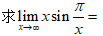（ ）
A.1
B.0
C.e
D.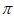9.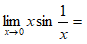（ ）
A.1
B.0
C.-1
D.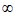10.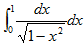等于（ ）
A.0
B.1
C.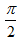D.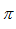11.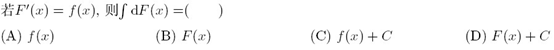A.(A)
B.(B)
C.(C)
D.(D)

12.设空间三点的坐标分别为M（1，1，1）、A（2，2，1）、B（2，1，2）。则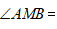（ ）
A.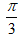B.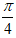C.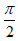D.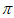13.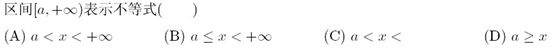A.(A)
B.(B)
C.(C)
D.(D)

14.若函数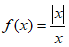，则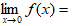（ 　）
A.0
B.-1
C.1
D.不存在

15.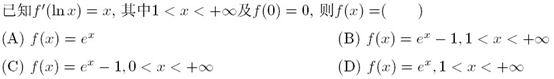A.(A)
B.(B)
C.(C)
D.(D)

16.以下函数中,哪个不是周期函数（ ）
A.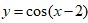B.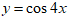C.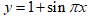D.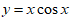17.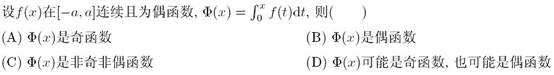A.(A)
B.(B)
C.(C)
D.(D)

18.当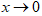时，变量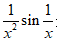是（ ）
A.无穷大
B.无穷小
C.有界的，但不是无穷小
D.无界的，但不是无穷大

19.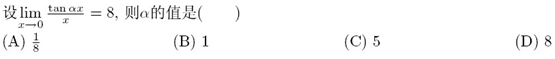A.(A)
B.(B)
C.(C)
D.(D)

20.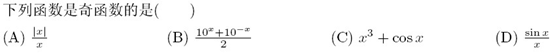A.(A)
B.(B)
C.(C)
D.(D)